Python机器学习基础教程

# 引子

``````import numpy as np
import matplotlib.pyplot as plt
import pandas as pd
import mglearn
import os
%matplotlib inline
``````

``````mglearn.plots.plot_animal_tree()
``````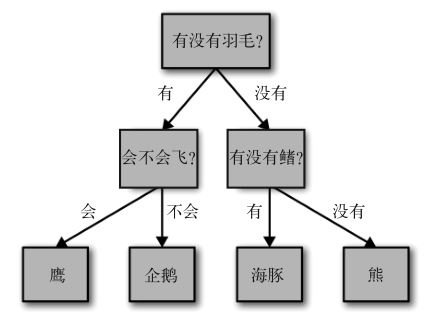# 1. 构造决策树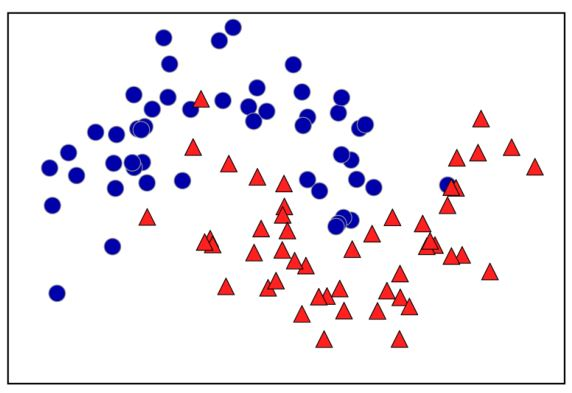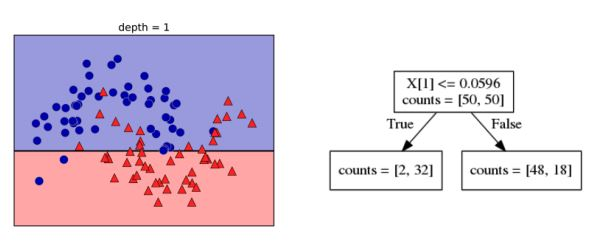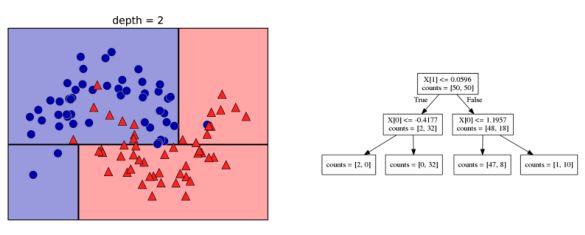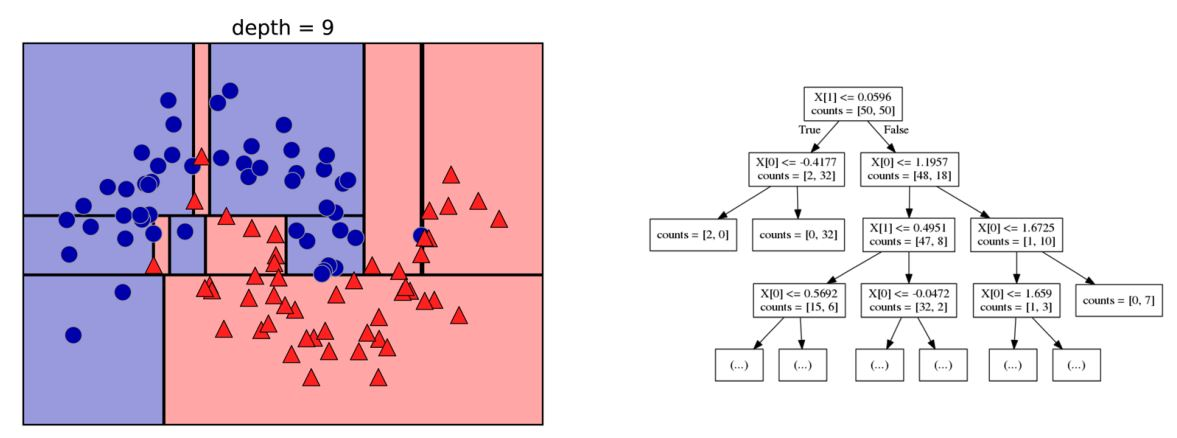# 2. 控制决策树的复杂度

scikit-learn 的决策树在 DecisionTreeRegressor 类和 DecisionTreeClassifier 类中实现。scikit-learn 只实现了预剪枝，没有实现后剪枝。

``````from sklearn.tree import DecisionTreeClassifier
from sklearn.model_selection import train_test_split

X_train, X_test, y_train, y_test = train_test_split(
cancer.data, cancer.target, stratify=cancer.target, random_state=42)
tree = DecisionTreeClassifier(random_state=0)
tree.fit(X_train, y_train)
print("Accuracy on training set: {:.3f}".format(tree.score(X_train, y_train)))
print("Accuracy on test set: {:.3f}".format(tree.score(X_test, y_test)))
``````

[out]：

Accuracy on training set: 1.000 Accuracy on test set: 0.937

``````tree=DecisionTreeClassifier(max_depth=4, random_state=0)
tree.fit(X_train, y_train)
print("Accuracy on training set: {:.3f}".format(tree.score(X_train, y_train)))
print("Accuracy on test set: {:.3f}".format(tree.score(X_test, y_test)))
``````

[out]：

Accuracy on training set: 0.988 Accuracy on test set: 0.951

# 3. 分析决策树

``````from sklearn.tree import export_graphviz
export_graphviz(tree, out_file="tree.dot", class_names=["malignant","benign"],
feature_names=cancer.feature_names, impurity=False, filled=True)
``````

``````import graphviz
with open("tree.dot") as f:
graphviz.Source(dot_graph)
``````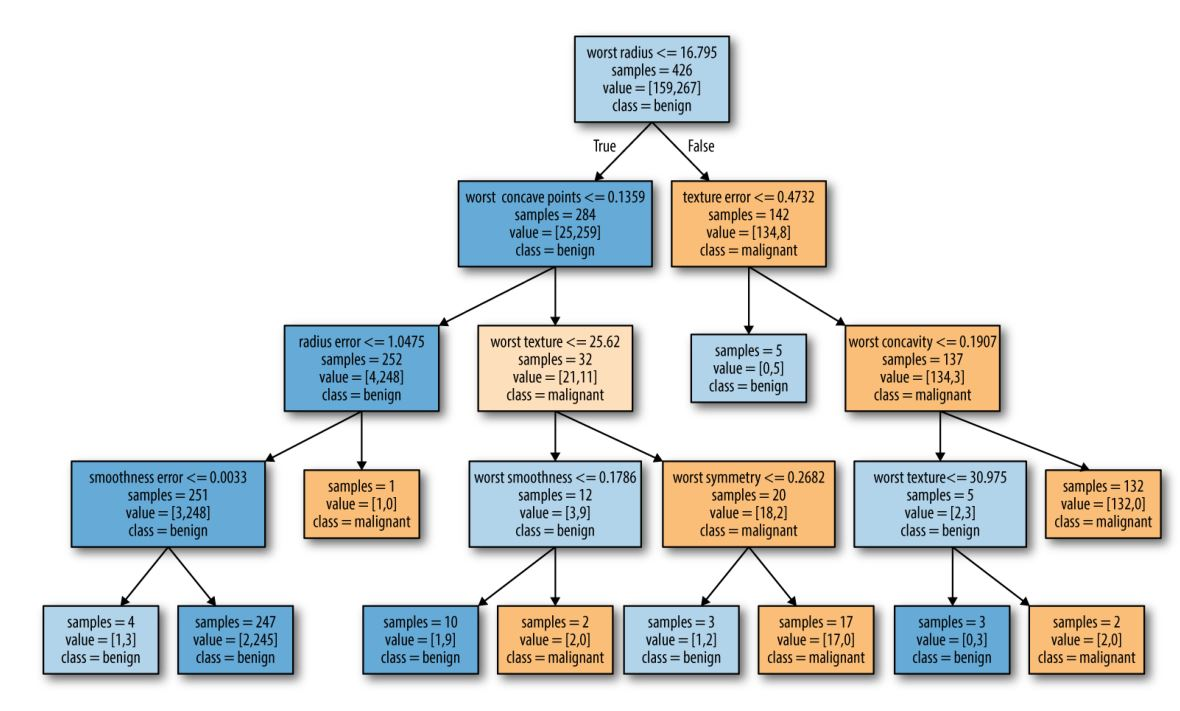# 4. 树的特征重要性

``````print("Feature importances:\n{}".format(tree.feature_importances_))
``````

[out]：

Feature importances: [ 0. 0. 0. 0. 0. 0. 0. 0. 0. 0. 0.01 0.048 0. 0. 0.002 0. 0. 0. 0. 0. 0.727 0.046

1. 0.014 0. 0.018 0.122 0.012 0. ]

``````def plot_feature_importance_cancer(model):
n_features = cancer.data.shape
plt.barh(range(n_features), model.feature_importances_, align='center')
plt.yticks(np.arange(n_features), cancer.feature_names)
plt.xlabel("Feature importance")
plt.ylabel("Feature")

plot_feature_importance_cancer(tree)
``````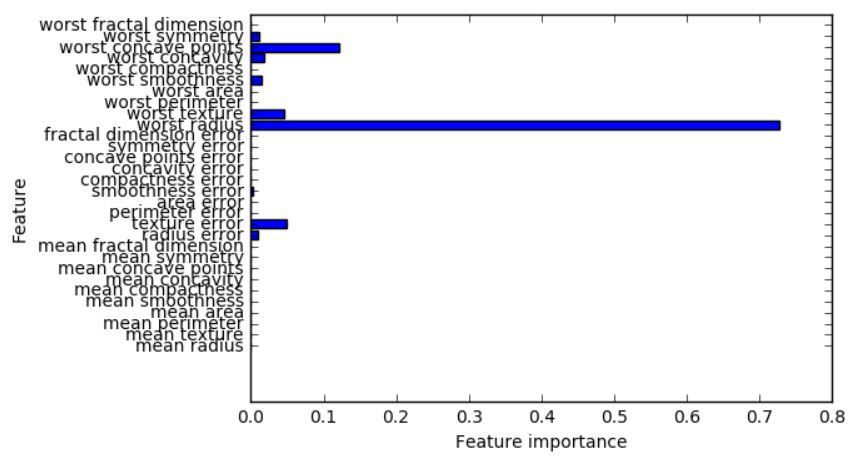``````tree = mglearn.plots.plot_tree_not_monotone()
``````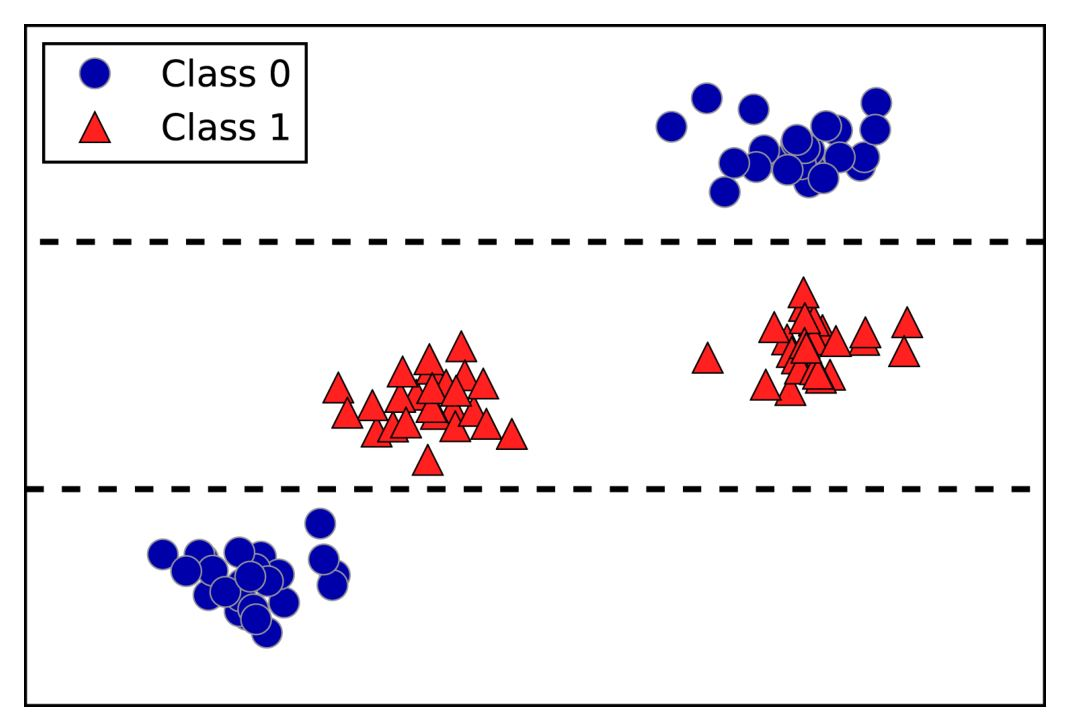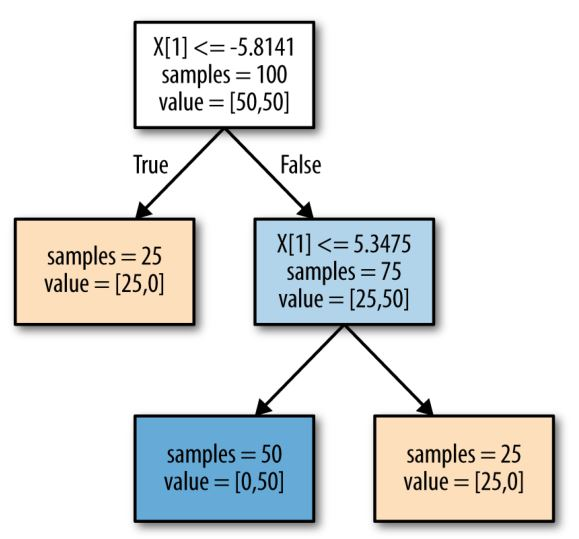``````import pandas as pd

plt.semilogy(ram_prices.date, ram_prices.price)
plt.xlabel('Year')
plt.ylabel("Pirce in \$/Mbyte")
``````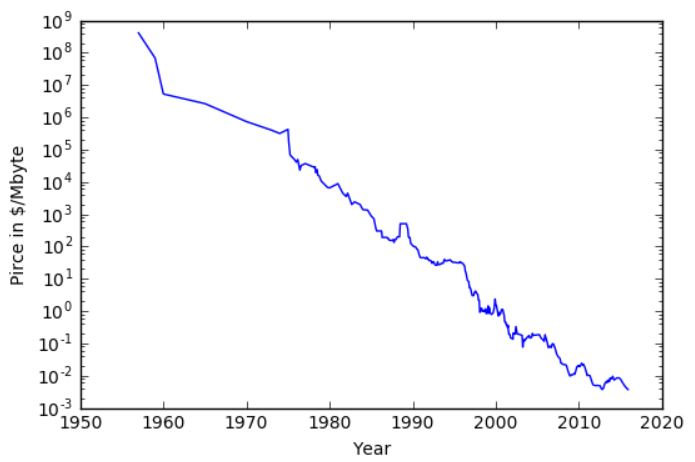``````from sklearn.tree import DecisionTreeRegressor
from sklearn.linear_model import LinearRegression
# 利用历史数据预测2000年后的价格
data_train = ram_prices[ram_prices.date < 2000]
data_test = ram_prices[ram_prices.date >= 2000]

# 基于日期来预测价格
X_train = data_train.date[:, np.newaxis]
# 我们利用对数变换得到数据和目标之间更简单的关系
y_train = np.log(data_train.price)

tree = DecisionTreeRegressor().fit(X_train, y_train)
linear_reg = LinearRegression().fit(X_train, y_train)

# 对所有数据进行预测
X_all = ram_prices.date[:, np.newaxis]

pred_tree = tree.predict(X_all)
pred_lr = linear_reg.predict(X_all)

# 对数变换逆运算
price_tree = np.exp(pred_tree)
price_lr = np.exp(pred_lr)
``````

``````plt.semilogy(data_train.date, data_train.price, label="Training data")
plt.semilogy(data_test.date, data_test.price, label="Test data")
plt.semilogy(ram_prices.date, price_tree, label="Tree prediction")
plt.semilogy(ram_prices.date, price_lr, label="Linear prediction")
plt.legend()
``````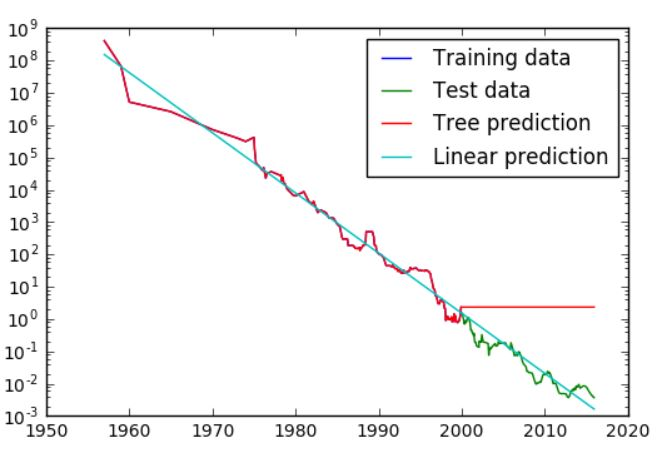# 5. 优点、缺点和参数技术小男生
4个月前
linux环境jdk环境变量配置
1：编辑系统配置文件vi/etc/profile2：按字母键i进入编辑模式，在最底部添加内容：JAVAHOME/opt/jdk1.8.0152CLASSPATH.:\$JAVAHOME/lib/dt.jar:\$JAVAHOME/lib/tools.jarPATH\$JAVAHOME/bin:\$PATH3：生效配置刚刚好
4个月前
css问题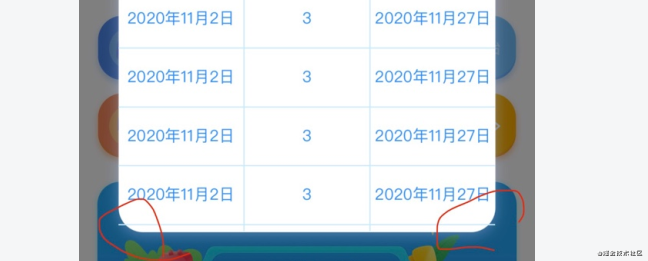blmius
1年前
MySQL:[Err] 1292 - Incorrect datetime value: ‘0000-00-00 00:00:00‘ for column ‘CREATE_TIME‘ at row 1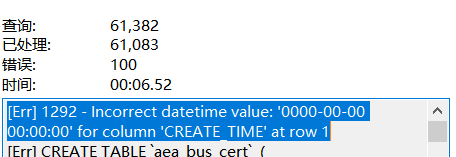晴空闲云
4个月前
css中box-sizing解放盒子实际宽高计算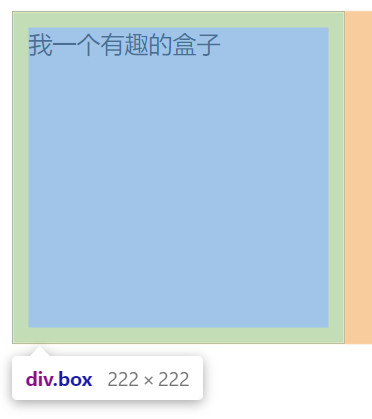艾木酱
3个月前

MemFireCloud是一款提供云数据库，用户可以创建云数据库，并对数据库进行管理，还可以对数据库进行备份操作。它还提供后端即服务，用户可以在1分钟内新建一个应用，使用自动生成的API和SDK，访问云数据库、对象存储、用户认证与授权等功能，可专Wesley13
1年前
MySQL查询按照指定规则排序
1.按照指定(单个)字段排序selectfromtable_nameorderiddesc;2.按照指定(多个)字段排序selectfromtable_nameorderiddesc,statusdesc;3.按照指定字段和规则排序selecStella981
1年前
Angular material matWesley13
1年前
MySQL部分从库上面因为大量的临时表tmp_table造成慢查询helloworld_34035044
6个月前

​在我们用皕杰报表工具设计填报报表时，如何在新增行里自动增加id呢？能新增整数排序id吗？目前可以在新增行里自动增加id，但只能用uuid函数增加UUID编码，不能新增整数排序id。uuid函数说明：获取一个UUID，可以在填报表中用来创建数据ID语法：uuid()或uuid(sep)参数说明：sep布尔值，生成的uuid中是否包含分隔符''，缺省为helloworld_28799839
4个月前

Javascript判断对象是否为空jsObject.keys(myObject).length0经常使用的三元运算我们经常遇到处理表格列状态字段如status的时候可以用到vueStella981
Lv1

1.7w

20

6